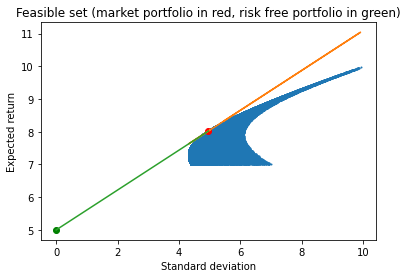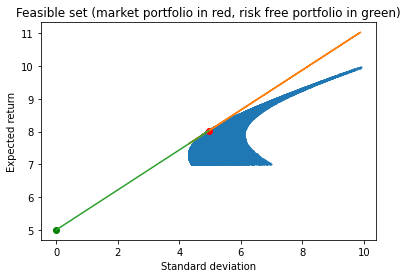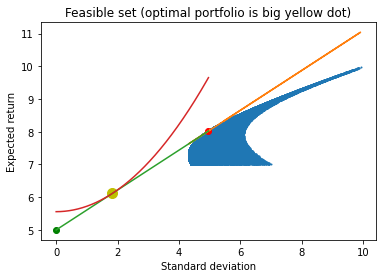# Classical portfolio theory¶

Consider a financial economy with three risky securities and one risk-free asset. The (percentage terms) expected returns of assets 1, 2, and 3 are $(7,10,7)$, respectively. The variance-covariance matrix of these returns (also in percentage terms) is: $$\Omega=\left[ \begin{array}{c c c} 49 & 10 & -10 \\ 10 & 100 & 10\\ -10 & 10& 49 \end{array} \right],$$

An investor cannot hold negative positions in any of those risky assets. The risk-free rate is $r_F=5\%$. The investor can borrow and lend however much she wants at that rate. Denote by $\alpha_i$ the non-negative share of her wealth she puts in security $i \in \{1,2,3\}$ while $\alpha_F$ is the (possibly negative) share of her wealth she invests in the risk-free asset. The investor has mean-variance preferences. Specifically, she seeks to maximize $$E(r_P) - \frac{\sigma\left(r_P\right)^2}{6}$$

where $$r_P=\alpha_F r_F+\alpha_1 r_1+\alpha_2 r_2+ \alpha_3 r_3$$

is her portfolio return, $E(r_P)$ is the expected value of that return, while $\sigma(r_P)$ is the standard deviation of that return. For future reference and in matrix notation: $$\sigma(r_P)=\sqrt{(\alpha_1,\alpha_2,\alpha_3) \Omega (\alpha_1,\alpha_2,\alpha_3)'}.$$

For more discussion, see chapter 2 of my notes. First we import the modules we will need.

In :
import pandas as pd # pandas is excellent for creating and manipulating dataframes, R-style
import numpy as np # great for array manipulations and simulations
# import scipy.linalg as la # for matrix algebra
import matplotlib.pyplot as plt #graphing module with matlab-like properties
%matplotlib inline


Now we start building the feasible set. This involves building lots of random portfolios and then calculating the expected return and standard deviation of the returns on those portfolios.

In :
from numpy import random # for random numbers below
import math

r=np.array([7,10,7]) # expected returns on risky assets
Omega=np.array([[49, 10, -10], [10, 100, 10], [-10 ,10 ,49]]) # variance-covariance matrix
rF=5 # risk-free rate

n=100000 # number of random portfolios

# and off we go
# using list comprehension to compactify everything
# list comprehension is awesome

# draws n random portfolios, all normalized so weights sum up to one
anowone=np.array([1/sum(row)*row for row in [np.random.rand(3) for i in range(n)]])
results=[[row @ r.T, math.sqrt(row @ Omega @ row.T)] for row in anowone] # expected returns and std
# combining these saves one trip through portfolios but now we unpack them
ret=np.array([item for item in results])
std=np.array([item for item in results])

sharpe=np.array([(ret[i]-rF)/std[i] for i in range(n)]) # sharpe ratio

# now we look for the approximate market portfolio
# it is the portfolio with the highest sharpe ratio

imax =np.argmax(sharpe) # this is the index of the portfolio with the highest sharpe ratio
market=anowone[imax]
sharpemarket=sharpe[imax]
retmarket=ret[imax]
stdmarket=std[imax]

fig, ax = plt.subplots()

plt.plot(std, ret, 'o',markersize=1) # the feasible set
plt.plot(stdmarket, retmarket, 'ro') # show market portfolio
plt.plot(0, rF, 'go') # show risk-free portfolio
plt.plot(std, sharpemarket*std + rF) # show efficient set in two steps
xnow=np.asarray([0,stdmarket]) #makes the multiplication below possible in Python syntax
plt.plot(xnow, sharpemarket*xnow + rF)

plt.title('Feasible set (market portfolio in red, risk free portfolio in green)')
ax.set_ylabel('Expected return')
ax.set_xlabel('Standard deviation')

print('The approximate market portfolio is: ', np.round(market,4))
print('The market return is: %.4f%%' %retmarket)
print('The std of the market return is: %.4f%%' %stdmarket)
print('The sharpe ratio of the market return is: %.4f' %sharpemarket)

The approximate market portfolio is:  [0.3304 0.3405 0.3291]
The market return is: 8.0215%
The std of the market return is: 4.9564%
The sharpe ratio of the market return is: 0.6096We can also find the market portfolio by solving $$\max_{(\alpha_1, \alpha_2,\alpha_3) \geq (0,0,0)} \frac{E(r_P)- r_F}{\sigma(r_P)}$$ subject to $$\alpha_1+ \alpha_2 + \alpha_3 =1.$$

In :
from scipy.optimize import minimize
from scipy.optimize import Bounds
from scipy.optimize import LinearConstraint

bounds=[(0,1),(0,1),(0,1)]
linear_constraint = LinearConstraint([[1, 1, 1]], 1,1)

def objective(x):
return -(x @ r.T-rF)/math.sqrt(x @ Omega @ x.T)

x0=np.array([1/2,1/4,1/4])

res = minimize(objective, x0, method='trust-constr',
constraints=[linear_constraint],
options={'verbose': 1}, bounds=bounds)

print('The market portfolio is: ',np.round(res.x,4))

print('The sharpe ratio of the market portfolio is %.4f' %-res.fun)


gtol termination condition is satisfied.
Number of iterations: 15, function evaluations: 28, CG iterations: 6, optimality: 6.31e-09, constraint violation: 0.00e+00, execution time: 0.05 s.
The market portfolio is:  [0.3297 0.3407 0.3297]
The sharpe ratio of the market portfolio is 0.6096


Now we can plot the CAPM line somewhat more exactly.

In :
fig, ax = plt.subplots()

marketexact=np.array([res.x,res.x,res.x])
retmarketexact=marketexact @ r.T
stdmarketexact=math.sqrt(marketexact @ Omega @ marketexact.T)

sharpemarketexact=(retmarketexact-5)/stdmarketexact

plt.plot(std, ret, 'o',markersize=1)
plt.plot(stdmarketexact, retmarketexact, 'ro')
plt.plot(0, rF, 'go')
plt.plot(std, sharpemarketexact*std + rF)
xtry=np.array([0,stdmarketexact])
plt.plot(xtry, sharpemarketexact*xtry + rF)

plt.title('Feasible set (market portfolio in red, risk free portfolio in green)')
ax.set_ylabel('Expected return')
ax.set_xlabel('Standard deviation')

Out:
Text(0.5, 0, 'Standard deviation')Now we find the optimal portfolio. We could once again use simulations and get as close to the answer as we want, but we will use optimization instead. We need to solve: $$\max_{\alpha_F \leq 1} E(r_P) - \frac{\sigma\left(r_P\right)^2}{6}$$ subject to: \begin{eqnarray*} E(r_P) & = & \alpha_F r_F +(1- \alpha_F) E(r_M) \\ \sigma(r_P) & = & (1-\alpha_F) \sigma(r_M) \end{eqnarray*} Traditional variational arguments show the solution to this to be:

$$\alpha_F=1-3\frac{E(r_M)-r_F}{\sigma(r_M)^2}\approx 0.63107.$$

Below we check with Python's optimization routine that it all works out.

In :
bounds=[(-1,1)]

def objective2(x):
Eret=x*5+(1-x)*retmarketexact
Stdret=(1-x)*stdmarketexact
return -Eret+Stdret**2/6

x0=0.75

res2 = minimize(objective2, x0, method='trust-constr',
options={'verbose': 1}, bounds=bounds)

print('The optimal risk-free share is: %.4f' %res2.x)
print('At the optimal, the expected return is: %.4f%%' %(res2.x*rF+(1-res2.x)*retmarketexact))
print('At the optimal, the standard deviation of the return is: %.4f%%' %((1-res2.x)*stdmarketexact))

gtol termination condition is satisfied.
Number of iterations: 14, function evaluations: 18, CG iterations: 8, optimality: 6.35e-09, constraint violation: 0.00e+00, execution time: 0.087 s.
The optimal risk-free share is: 0.6311
At the optimal, the expected return is: 6.1149%
At the optimal, the standard deviation of the return is: 1.8289%


Let's now locate this portfolio on our graph and plot the indifference curve that runs through it to get a nice tangency.

In :
fig, ax = plt.subplots()

marketexact=np.array([res.x,res.x,res.x])
retmarketexact=marketexact @ r.T
stdmarketexact=math.sqrt(marketexact @ Omega @ marketexact.T)

sharpemarketexact=(retmarketexact-rF)/stdmarketexact

plt.plot(std, ret, 'o',markersize=1)
plt.plot(stdmarketexact, retmarketexact, 'ro')
plt.plot((1-res2.x)*stdmarketexact, res2.x*5+(1-res2.x)*retmarketexact, 'yo',markersize=10)
plt.plot(0, rF, 'go')
plt.plot(std, sharpemarketexact*std + rF)
xtry=np.array([0,stdmarketexact])
plt.plot(xtry, sharpemarketexact*xtry + rF)

plt.title('Feasible set (optimal portfolio is big yellow dot)')

# now for good measure we add the indifference curve that runs thru the optimal portfolio

Ustar=res2.x*rF+(1-res2.x)*retmarketexact-((1-res2.x)*stdmarketexact)**2/6 # the value of the objective at the optimal portfolio
stdopt=np.linspace(0, stdmarketexact, num=500)
plt.plot(stdopt, Ustar + stdopt**2/6)

ax.set_ylabel('Expected return')
ax.set_xlabel('Standard deviation')

Out:
Text(0.5, 0, 'Standard deviation')